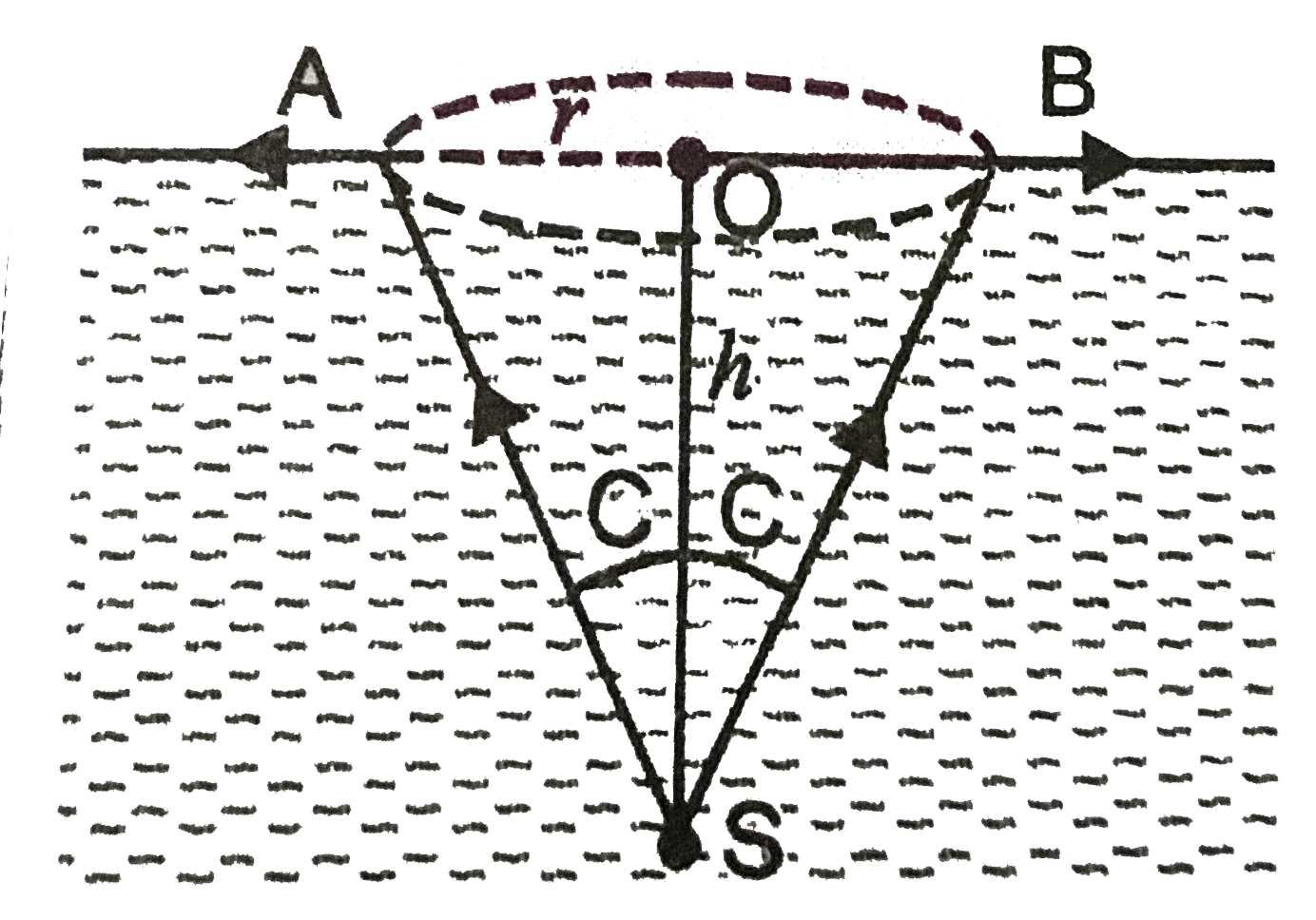# A point source of light S is placed at the bottom of a vessel containing a liquid of refractive index 5//3. A person is viewing the source from ab

499 views
in Physics
closed
A point source of light S is placed at the bottom of a vessel containing a liquid of refractive index 5//3. A person is viewing the source from above the surface. There is an opaque disc of radius 1 cm floating on the surface. The centre of disc lies vertically above the source O. The liquid from the vessel is gradually drained out through a tap. What is the maximum height of the liquid for which the source cannot be seen at all.

by (86.1k points)
selected

In , let OS = h be the max. height of liquid above the source for which the source cannot be seen at all.
:. /_OSA = C
Any other ray from S will be totally internally reflected because in that case, angle of incidence will be greater them the critical angle.
In Delta OSA, sin C = (OA)/(SA) = (r)/(sqrt(r^2 + h^2)) = (1)/(mu)
or (1)/(mu^2) = (r^2)/(r^2 + h^2)
r^2 + h^2 = mu^2 r^2
h^2 = r^2 (mu^2 - 1)
h = r sqrt(mu^(2) - 1) = 1 sqrt((5//3)^(2) - 1)
h = (4)/(3) = 1.33 cm..